# Title 40

## SECTION 53.63

### 53.63 Test procedure: Wind tunnel inlet aspiration test.

§ 53.63 Test procedure: Wind tunnel inlet aspiration test.

(a) Overview. This test applies to a candidate sampler which differs from the reference method sampler only with respect to the design of the inlet. The purpose of this test is to ensure that the aspiration of a Class II candidate sampler is such that it representatively extracts an ambient aerosol at elevated wind speeds. This wind tunnel test uses a single-sized, liquid aerosol in conjunction with wind speeds of 2 km/hr and 24 km/hr. The test atmosphere concentration is alternately measured with the candidate sampler and a reference method device, both of which are operated without the 2.5-micron fractionation device installed. The test conditions are summarized in table F-2 of this subpart (under the heading of “wind tunnel inlet aspiration test”). The candidate sampler must meet or exceed the acceptance criteria given in table F-1 of this subpart.

(b) Technical definition. Relative aspiration is the ratio (expressed as a percentage) of the aerosol mass concentration measured by the candidate sampler to that measured by a reference method sampler.

(c) Facilities and equipment required. The facilities and equipment are identical to those required for the full wind tunnel test (§ 53.62(c)).

(d) Setup. The candidate and reference method samplers shall be operated with the PM 2.5 fractionation device removed from the flow path throughout this entire test procedure. Modifications to accommodate this requirement shall be limited to removal of the fractionator and insertion of the filter holder directly into the downtube of the inlet.

(e) Test procedure - (1) Establish the wind tunnel test atmosphere. Follow the procedures in § 53.62(d)(1) through (d)(4) to establish a test atmosphere for one of the two wind speeds specified in table F-2 of this subpart.

(2) Measure the aerosol concentration with the reference sampler. (i) Install the reference sampler (or portion thereof) in the wind tunnel with the sampler inlet opening centered in the sampling zone. To meet the maximum blockage limit of § 53.62(c)(1) or for convenience, part of the test sampler may be positioned external to the wind tunnel provided that neither the geometry of the sampler nor the length of any connecting tube or pipe is altered. Collect particles for a time period such that the relative error of the measured concentration is less than 5.0 percent.

(ii) Determine the quantity of material collected with the reference method sampler using a calibrated fluorometer. Calculate and record the mass concentration as:

Equation 15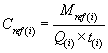where: i = replicate number; Mref = mass of material collected with the reference method sampler; Q = reference method sampler volumetric flow rate; and t = sampling time.

(iii) Remove the reference method sampler from the tunnel.

(3) Measure the aerosol concentration with the candidate sampler. (i) Install the candidate sampler (or portion thereof) in the wind tunnel with the sampler inlet centered in the sampling zone. To meet the maximum blockage limit of § 53.62(c)(1) or for convenience, part of the test sampler may be positioned external to the wind tunnel provided that neither the geometry of the sampler nor the length of any connecting tube or pipe is altered. Collect particles for a time period such that the relative error of the measured concentration is less than 5.0 percent.

(ii) Determine the quantity of material collected with the candidate sampler using a calibrated fluorometer. Calculate and record the mass concentration as:

Equation 16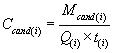where: i = replicate number; Mcand = mass of material collected with the candidate sampler; Q = candidate sampler volumetric flow rate; and t = sampling time.

(iii) Remove the candidate sampler from the wind tunnel.

(4) Repeat steps in paragraphs (d) (2) and (d)(3) of this section. Alternately measure the tunnel concentration with the reference sampler and the candidate sampler until four reference sampler and three candidate sampler measurements of the wind tunnel concentration are obtained.

(5) Calculations. (i) Calculate and record aspiration ratio for each candidate sampler run as:

Equation 17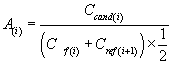where: i = replicate number.

(ii) Calculate and record the mean aspiration ratio as:

Equation 18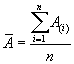where: i = replicate number; and n = total number of measurements of aspiration ratio.

(iii) Precision of the aspiration ratio. (A) Calculate and record the precision of the aspiration ratio measurements as the coefficient of variation as:

Equation 19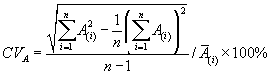where: i = replicate number; and n = total number of measurements of aspiration ratio.

(B) If the value of CVA exceeds 10 percent, the entire test procedure must be repeated.

(f) Evaluation of test results. The candidate method passes the inlet aspiration test if all values of A meet the acceptance criteria specified in table F-1 of this subpart.